To prove relation reflexive, transitive, symmetric and equivalent

Chapter 1 Class 12 Relation and Functions
Concept wise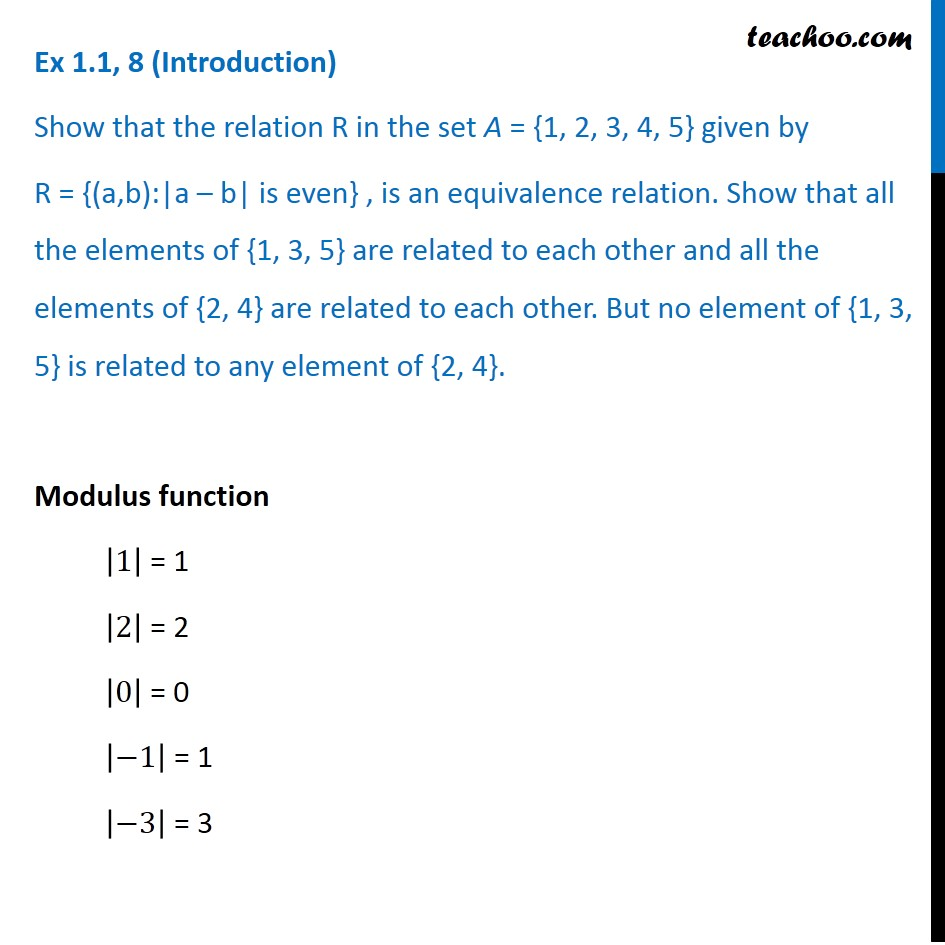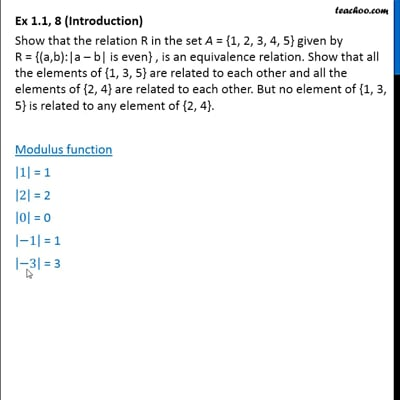This video is only available for Teachoo black users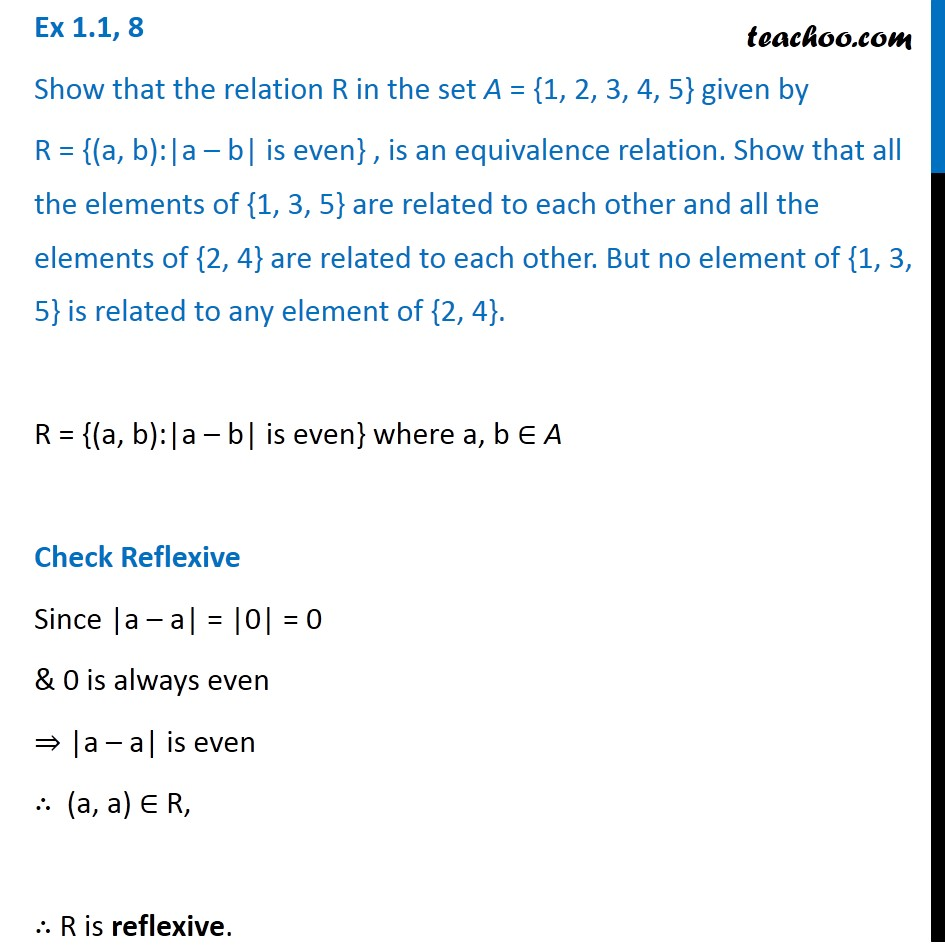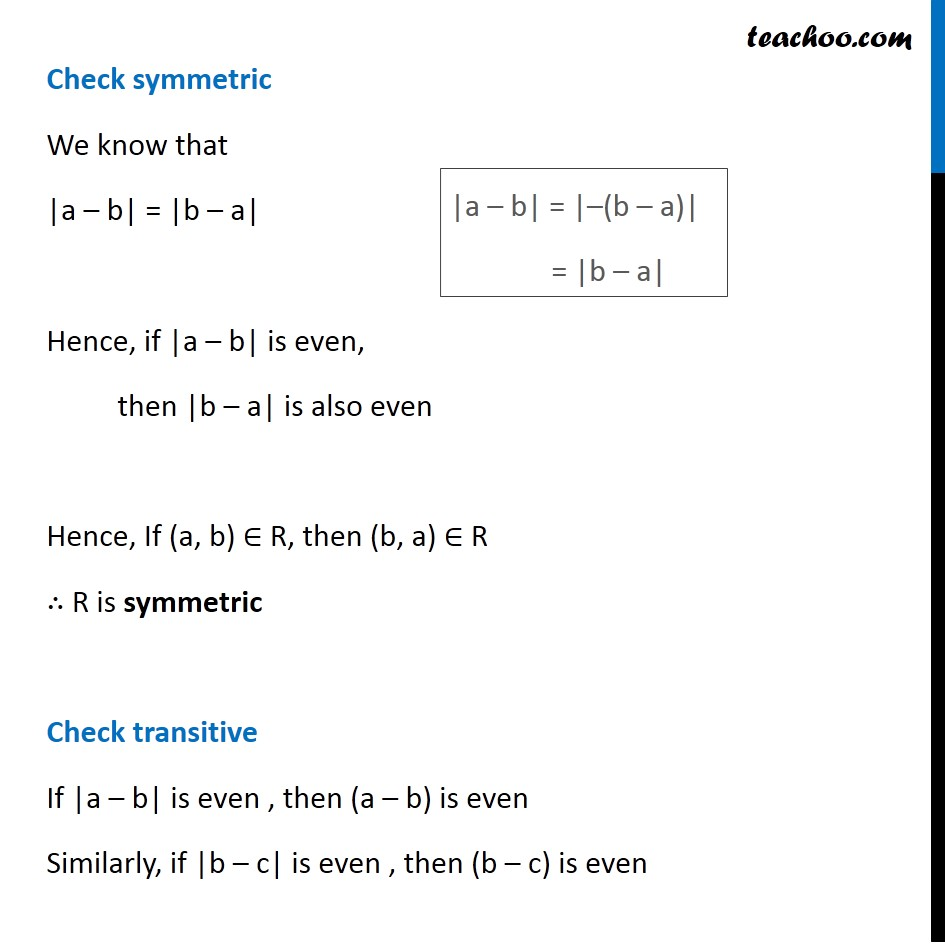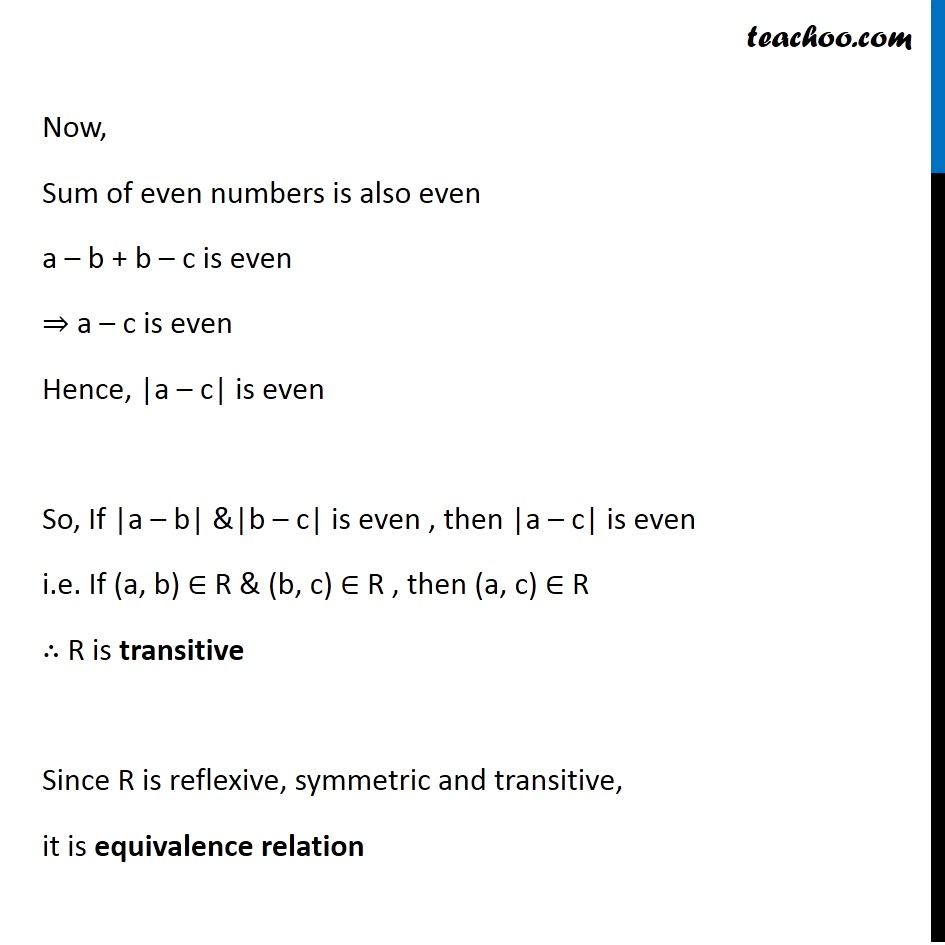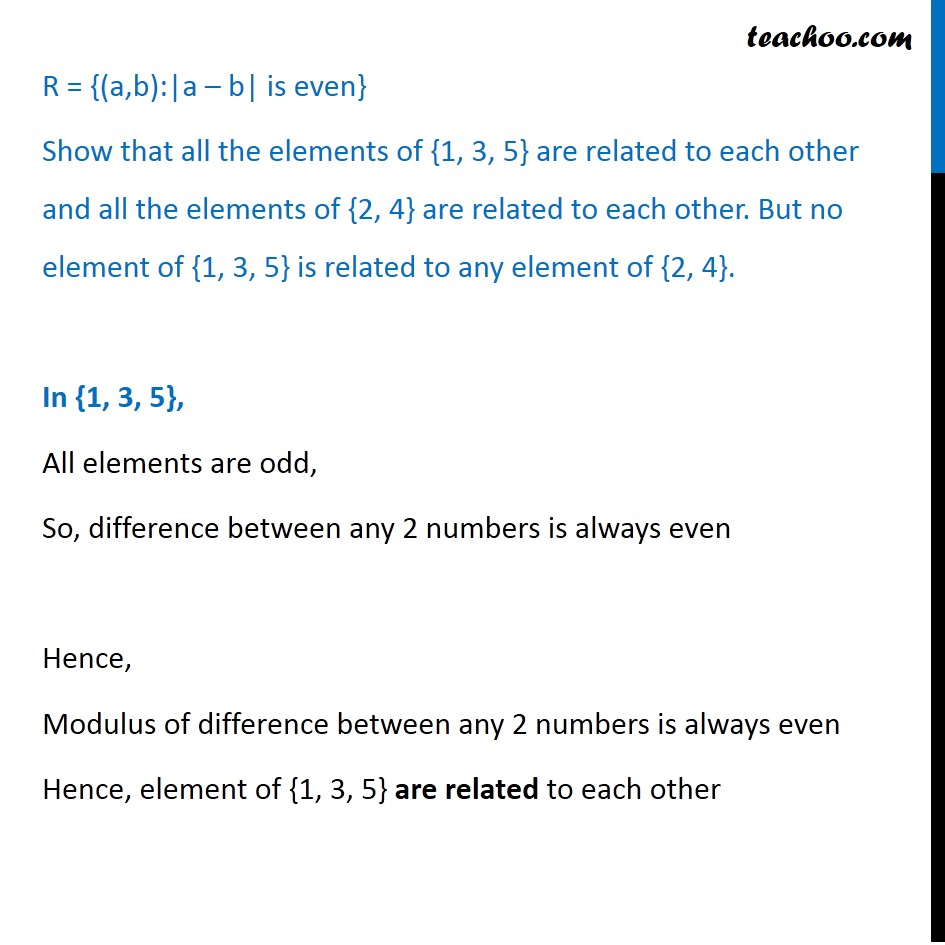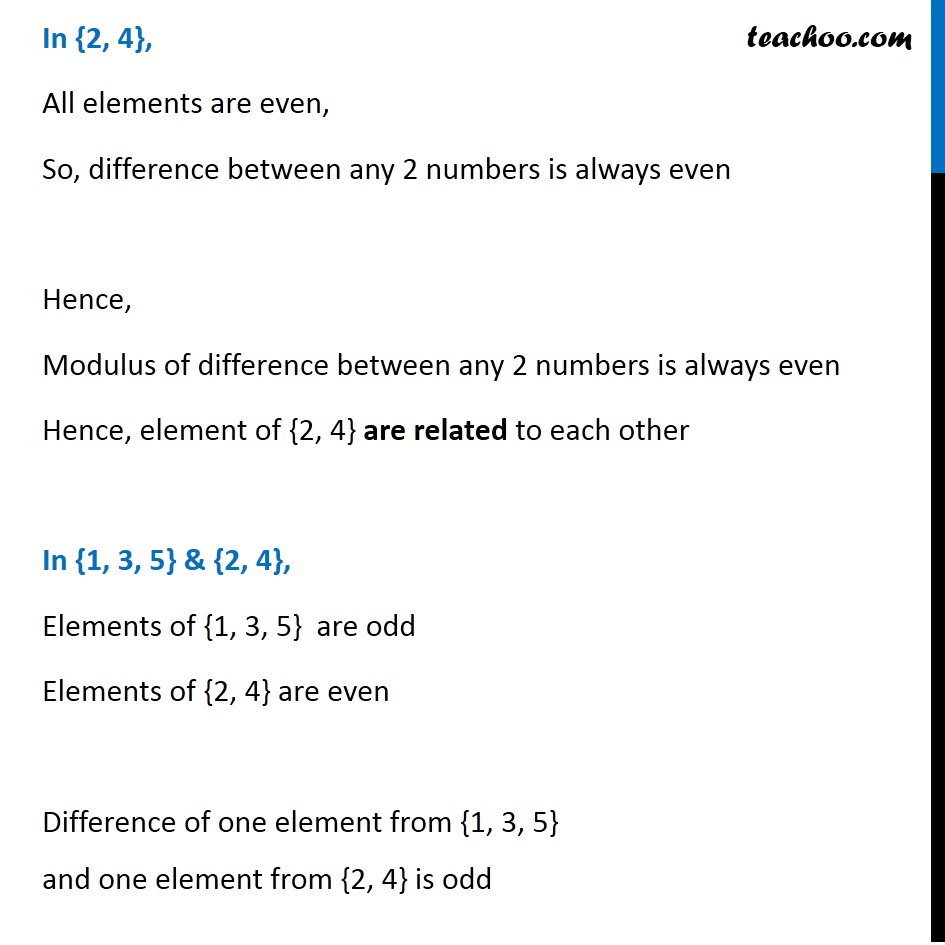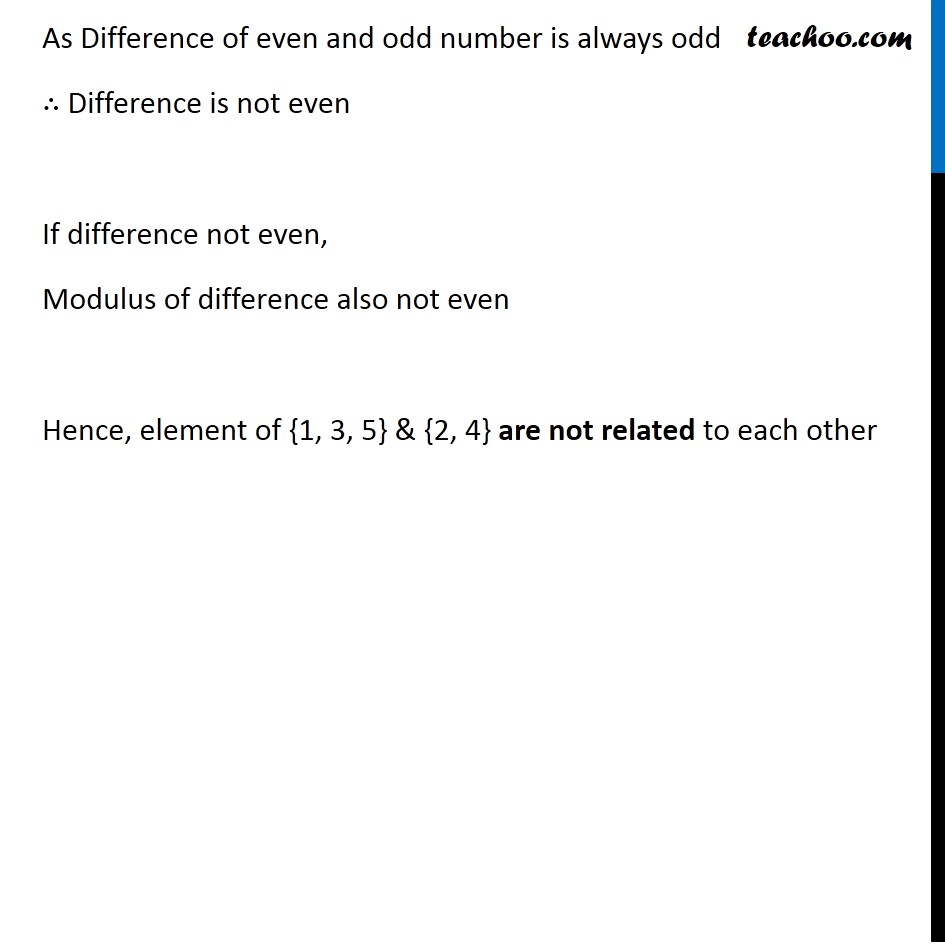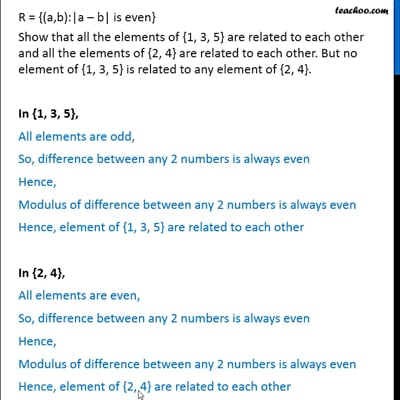This video is only available for Teachoo black users

Introducing your new favourite teacher - Teachoo Black, at only ₹83 per month

### Transcript

Ex 1.1, 8 (Introduction) Show that the relation R in the set A = {1, 2, 3, 4, 5} given by R = {(a,b):|a – b| is even} , is an equivalence relation. Show that all the elements of {1, 3, 5} are related to each other and all the elements of {2, 4} are related to each other. But no element of {1, 3, 5} is related to any element of {2, 4}. Modulus function |1| = 1 |2| = 2 |0| = 0 |−1| = 1 |−3| = 3 Ex 1.1, 8 Show that the relation R in the set A = {1, 2, 3, 4, 5} given by R = {(a, b):|a – b| is even} , is an equivalence relation. Show that all the elements of {1, 3, 5} are related to each other and all the elements of {2, 4} are related to each other. But no element of {1, 3, 5} is related to any element of {2, 4}. R = {(a, b):|a – b| is even} where a, b ∈ A Check Reflexive Since |a – a| = |0| = 0 & 0 is always even ⇒ |a – a| is even ∴ (a, a) ∈ R, ∴ R is reflexive. Check symmetric We know that |a – b| = |b – a| Hence, if |a – b| is even, then |b – a| is also even Hence, If (a, b) ∈ R, then (b, a) ∈ R ∴ R is symmetric Check transitive If |a – b| is even , then (a – b) is even Similarly, if |b – c| is even , then (b – c) is even |a – b| = |–(b – a)| = |b – a| Now, Sum of even numbers is also even a – b + b – c is even ⇒ a – c is even Hence, |a – c| is even So, If |a – b| &|b – c| is even , then |a – c| is even i.e. If (a, b) ∈ R & (b, c) ∈ R , then (a, c) ∈ R ∴ R is transitive Since R is reflexive, symmetric and transitive, it is equivalence relation R = {(a,b):|a – b| is even} Show that all the elements of {1, 3, 5} are related to each other and all the elements of {2, 4} are related to each other. But no element of {1, 3, 5} is related to any element of {2, 4}. In {1, 3, 5}, All elements are odd, So, difference between any 2 numbers is always even Hence, Modulus of difference between any 2 numbers is always even Hence, element of {1, 3, 5} are related to each other In {2, 4}, All elements are even, So, difference between any 2 numbers is always even Hence, Modulus of difference between any 2 numbers is always even Hence, element of {2, 4} are related to each other In {1, 3, 5} & {2, 4}, Elements of {1, 3, 5} are odd Elements of {2, 4} are even Difference of one element from {1, 3, 5} and one element from {2, 4} is odd As Difference of even and odd number is always odd ∴ Difference is not even If difference not even, Modulus of difference also not even Hence, element of {1, 3, 5} & {2, 4} are not related to each other